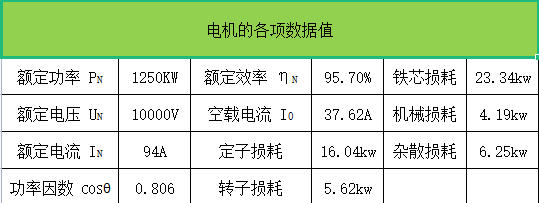# 10kv电动机就地无功补偿容量计算Q=PN *[tan(cos0.806)-1-tan(cos0.92)-1]=1250X0.308=385kvar

1.2电动机在额定工况时的损耗计算

∑PN=定子损耗+转子损耗+铁芯损耗+机械损耗+杂散损耗=16.04+5.62+23.34+4.19+6.25=55.44KW

P0=铁芯损耗+机械损耗 =23.34+4.19=27.53KW

PB=总损耗-固定损耗 =55.44-27.53=27.91KW

1.3 电动机实际输出功率和负载率计算

P2=PN *(I22-I02)/(IN2-I02)=1250*(712-37.642)/(942-37.642)=875KW

β=P2/PN=875/1250=0.7（负载率：以上百分数表示的负载系数，这里为0.7）

1.4 电动机在负载率β=70%，P2=875kw时电动机的各项参数计算和补偿容量的计算：

∑P=K2*PB+P0=41.2KW

P1=P2+∑P =875+41.2=916KW

Q1=P1*[tan(cos0.745)-1-tan(cos0.92)-1] =916*0.469=430kvar

1.5 分析原因

Uc2=UN/(1-6%)=10/0.94=10.64KV

Qc=(Uc22*Q)/Uc2=(10.642*400)/112=374kvar

Q2=电容器实际输出容量-电抗器容量 =374-24=350kvar